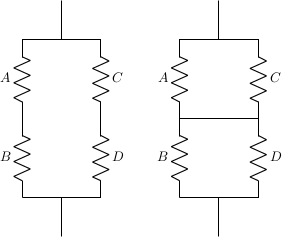# Lehman’s inequality, circuits, and LaTeX

Let A, B, C, and D be positive numbers. Then Lehman’s inequality says## Proof by circuit

This inequality can be proved analytically, but Lehman’s proof is interesting because he uses electrical circuits .

Let A, B, C, and D be the resistances of resistors arranges as in the circuit on the left.Resistors R1 and R2 in series have a combined resistance ofand the same resistors in parallel have a combined resistance ofThis means the circuit on the left has combined resistanceThe resistance of the circuit on the right isAdding a short cannot increase resistance, so the resistance of the circuit on the right must be the same or lower than the resistance of the one on the left. Therefore## Drawing circuits in LaTeX

I drew the circuits above using the circuitikz package in LaTeX. Here’s the code to draw the circuit on the left.

    \documentclass{article}

\usepackage{tikz}
\usepackage{circuitikz}

\begin{document}

\begin{figure}[h!]
\begin{center}
\begin{circuitikz}
\draw (0,0)
to[R=$B$] (0,2)
to[R=$A$] (0,4)
to[short] (2,4)
to[R=$C$] (2,2)
to[R=$D$] (2,0)
to[short] (0,0);
\draw (1,4)
to[short] (1, 5);
\draw (1, 0)
to[short] (1, -1);
%\draw (0, 2)
%to[short] (2, 2);
\end{circuitikz}
\end{center}
\end{figure}

\end{document}


The code to draw the second circuit removes the %’s commenting out the code that draws the short between the two parallel arms of the circuit.

 Alfred Lehman proves a more general result using circuits in SIAM Review, Vol. 4, No. 2 (April 1962) pp. 150–151. Fazlollah Reza gives a purely mathematical proof on pages 151 and 152 of the same journal.

## One thought on “Lehman’s inequality, circuits, and LaTeX”

1. “Proof by circuit” indeed!

While taking my first analog circuit theory class in 1981, while covering KCL and KVL I remember the professor starting to share some equivalences from other circuit analysis approaches, and then some ways KCL and KVL could be used to do proofs in other domains.

Unfortunately, the moment I heard “This won’t be on the exam.”, I completely tuned out and have zero memory of what the examples were.